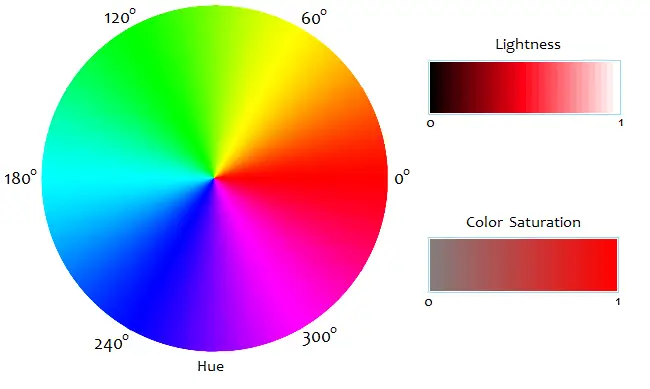# RGB to HSL, HSL to RGB Conversion Calculator

RGB/HSL Conversion Calculator
RGB to HSL

HSL to RGB

Color
RGB values range from 0 to 255. Hue ranges from 0° to 360°. Saturation ranges from 0 to 1. Lightness ranges from 0 to 1.

The RGB color model describes a color in terms of the amount of red, green, and blue light it contains. The three values of red, green, and blue range from 0 to 255, with white defined as (255, 255, 255), black as (0, 0, 0), red as (255, 0, 0), green as (0, 255, 0), and blue as (0, 0, 255).

Another color system is the HSL model, which describes a color in terms of its Hue, Saturation, and Lightness. The hue of a color is the angle measure of its position on a color wheel, where 0° = red, 60° = yellow, 120° = green, 1800° = cyan, 240° = blue, 300° = magenta, and all the intermediate hues are represented by intermediate angles.

The saturation of a color is its closeness to neutral colors. Brighter, purer colors have higher saturation values; muted, grayer colors have lower saturation levels. Colors close to white and black also saturation levels near 0. The mathematical equation for saturation varies by color model; see HSI and HSV to compare.

Lightness (not to be confused with luma and luminescence in other color schemes) is just what it sounds like; it describes how light or dark a color is. In the HSL system, lightness ranges from 0 to 1. White has a lightness value of 1 or 100%, black is 0 or 0%, while pure colors at full saturation have a lightness value of 0.5 or 50%.### Equations to Convert RGB to HSL

To compute the lightness and saturation from the values of R, G, and B, first define three numbers M, m, and d as follows:

M = max{R, G, B}
m = min{R, G, B}
d = (M - m)/255.

The lightness, L, of a color is given by the equation

L = [½(M + m)]/255 = (M + m)/510.

The saturation, S, is given by the formula

S = d/[1 - |2L-1|]        if L > 0
S = 0                         if L = 0.

The angle measures for H are calculated with the equations

H = cos-1[ (R - ½G - ½B)/√R² + G² + B² - RG - RB - GB ]            if G ≥ B, or
H = 360 - cos-1[ (R - ½G - ½B)/√R² + G² + B² - RG - RB - GB ]    if B > G,

where the cos-1 is in degrees, not radians. For neutral colors white, gray, and black, the angle measure is defined to be 0° for convenience and consistency.

### Equations to Convert HSL to RGB

To work backwards and reconstruct RGB values from the HSL values, you first compute d and m with the equations

d = S(1 - |2L-1|)
m = 255(L - ½d).

Now define another number, x, with the equation

x = d[1 - |(H/60)mod_2 - 1|],

where mod_2 means division modulo 2. For example, if H = 213, then (H/60)mod_2 = (3.55)mod_2 = 1.55. Modulo 2 division returns the remainder of the quantity when you divide it by 2.

Now you can find the values of R, G, and B according to the angle range of H. Whenever 0 ≤ H < 60, you have

R = 255d + m
G = 255x + m
B = m.

If 60 ≤ H < 120,

R = 255x + m
G = 255d + m
B = m.

If 120 ≤ H < 180,

R = m
G = 255d + m
B = 255x + m.

When 180 ≤ H < 240,

R = m
G = 255x + m
B = 255d + m.

When 240 ≤ H < 300,

R = 255x + m
G = m
B = 255d + m.

And if 300 ≤ H < 360,

R = 255d + m
G = m
B = 255x + m.